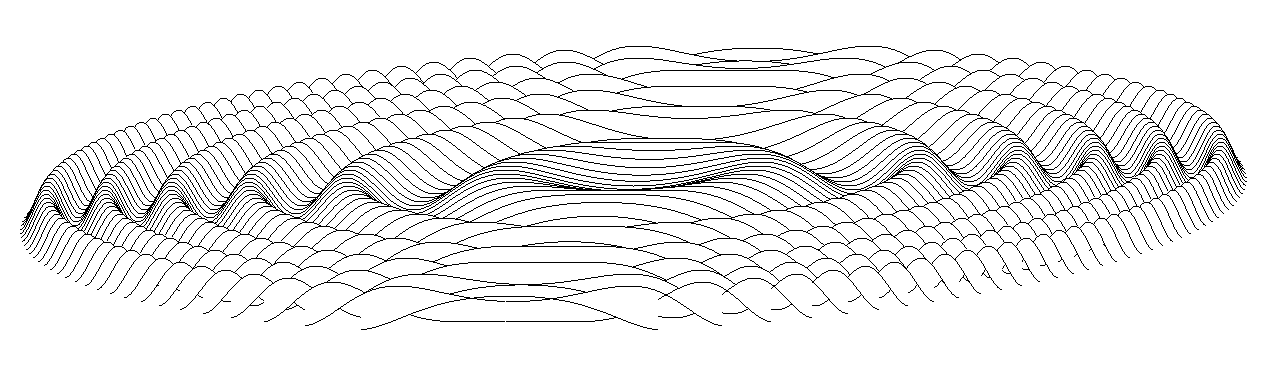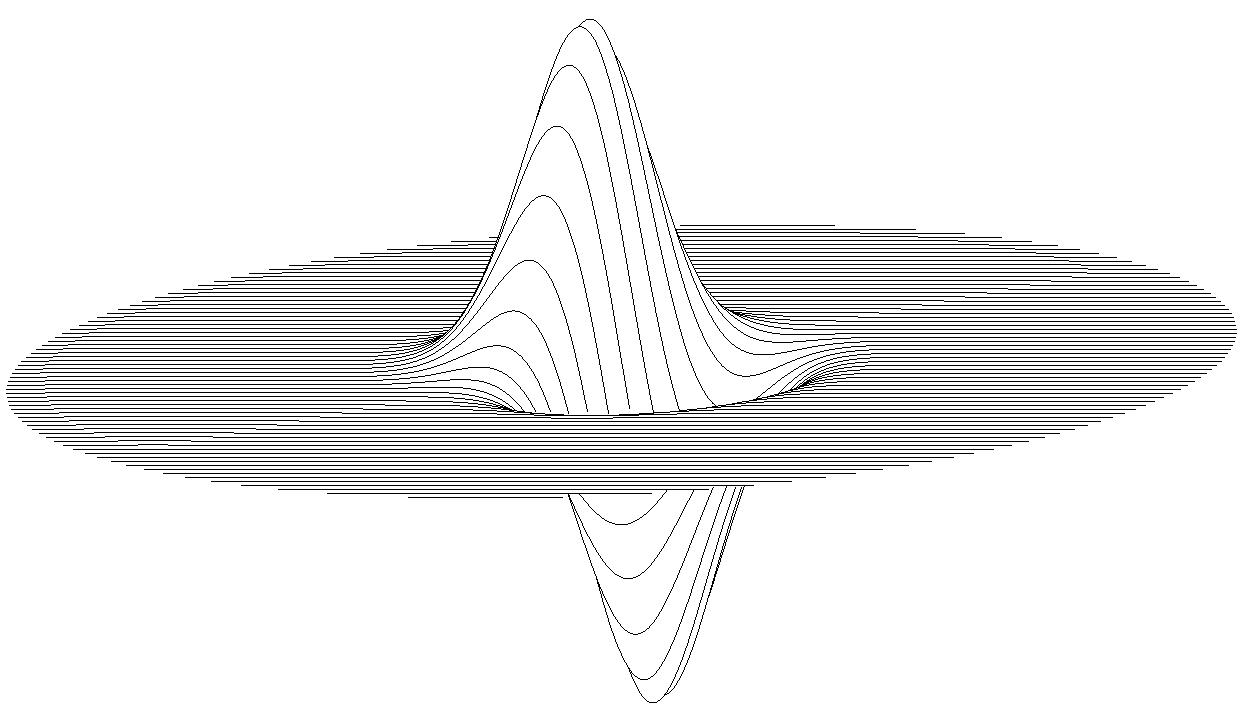For most people nowadays the first programming language is python or javascript. Once upon a time that was not the case, and probably BASIC was the one where most millennials got their feet wet.

That actually never happened for me – I barely knew what “programming” meant before my first university courses, which technically makes Assembly and C my first programming languages. Last week I was skimming through an undergrad level Calculus book (old, but still used by students in Italy), and there I found a juicy chapter on plotting mathematical functions (with 1 or 2 variables) with.. BASIC.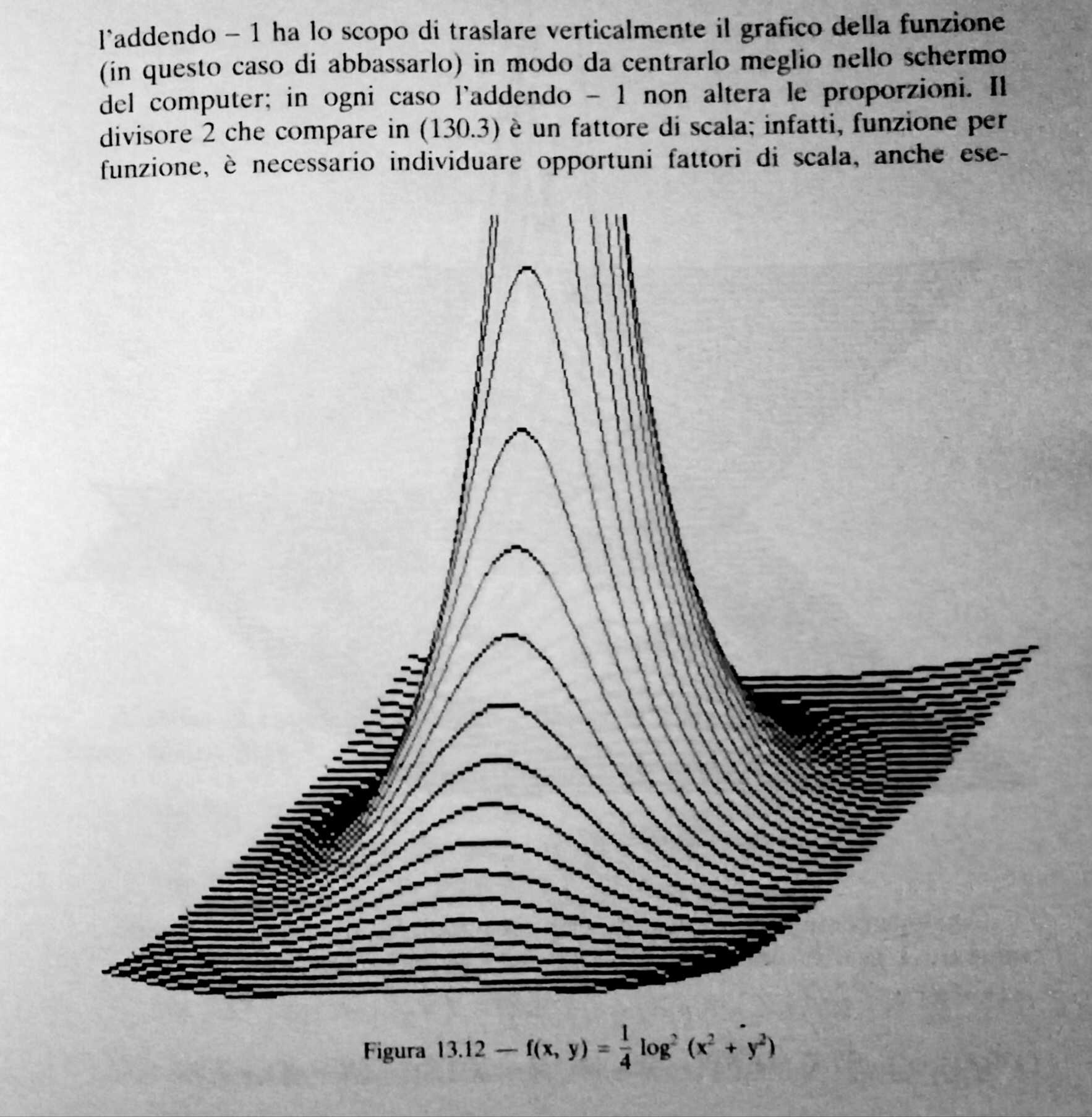I was rather intrigued by the nice looking plots, and how the code actually looked pretty terse! I had to try and replicate those results.

BASIC is more a family of languages than a single one. I picked one that I could easily install on ubuntu (sudo apt install yabasic) and was good to go. This turned out to be a slightly more modern dialect than the one in the book, but the gist is the same.

### 2d plots

First thing: plotting single-variable functions.

W = 600
H = 600
OPEN WINDOW W,H

X0 = W/2
Y0 = H/2
LINE 0,Y0,W,Y0 //X-AXIS
LINE X0,0,X0,H //Y-AXIS

SUB FUN(X)
RETURN 200*SIN((X/50))
END SUB

FOR X = 0 TO W
Y = -FUN(X - X0) + Y0 //invert y coordinate and center in Y0
LINE X,Y
NEXT X


In the first block I’m opening a new window with size (W,H). Then I’m plotting the x and y axis in the middle of the window. FUN is the function I want to plot. Finally, a for loop where I need to convert the y value in the actual “window” coordinate (in the output window, the point (0,0) is the upper left corner). The LINE X,Y instruction does the heavy lifting here: if no LINE was previously called, the current point is set to X,Y; otherwise, it draws a line from the previous point to X,Y. Pretty nifty and concise.

This is the glorious result: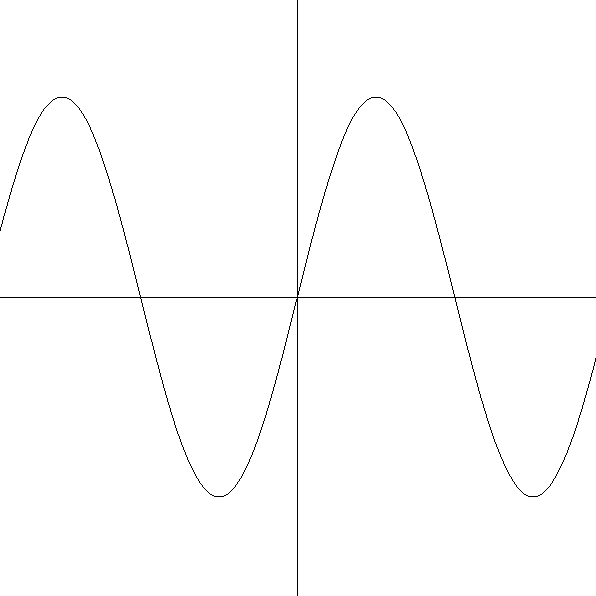Note that, in the function definition, one needs to make sure the plot can actually be visualized in the window in a decent way – meaning, we need to scale and/or translate it so the window contains something meaningful.

Another easy trick shown in the book is how we can plot a filled curve, where for each X we draw a line between (X,Y) and the x-axis:

FOR X = 0 TO W
Y = -FUN(X - X0) + Y0 //invert y coordinate and center in Y0
LINE X,Y0,X,Y
NEXT X


With $y = sin(1/x)$ (and some appropriate scaling) this is what you get: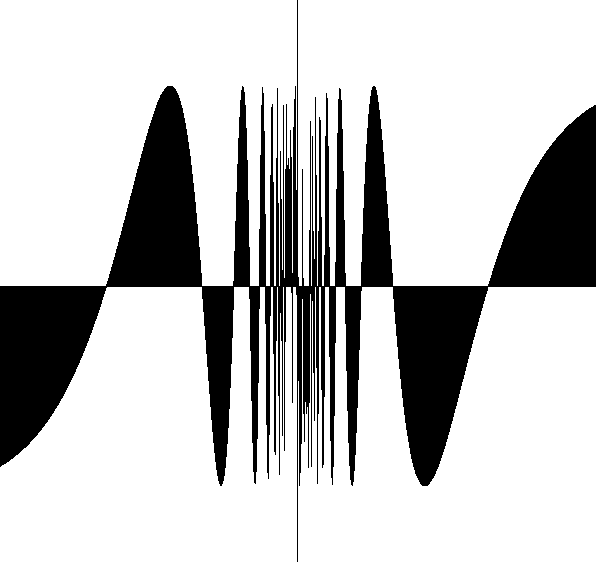### 3d plots

Plotting 2-variables functions in a 3d space is a bit trickier. Given $Z=F(X,Y)$, one needs to transform the $(X,Y,Z)$ point into $(U,V)$-coordinates, that is, the coordinates relative to the window. Let’s assume that $X$ is the axis which is normal to the screen, while $Z$ is the vertical axis and $Y$ the lateral axis. The idea is to draw lines (“slices” of the surface) connecting consecutive points with constant $X$. To give depth and obtain a 3d-ish view, each line is shifted up and right by a small amount of pixels (SHIFT).

W = 1400
H = 1000
OPEN WINDOW W,H
U0 = W/2
V0 = H/2

SUB FUN(X,Y)
//Define function here
END SUB

SHIFT = 4
X = 0
NUM_LINES = 80
FOR I=-NUM_LINES/2 TO NUM_LINES //number of lines to plot
X = I*(W/NUM_LINES)
NEW CURVE
FOR Y=-W TO W
Z = FUN(X,Y)
U = Y + U0 + I*SHIFT
V = -Z + V0 - I*SHIFT
LINE U,V
NEXT Y
NEXT I


With the NEW CURVE instruction, we stop keeping track of the latest point and a new LINE is started. This code returns a 3d plot, but the result is a bit of a mess even for a simple function like $z = sin(y)$:To avoid this issue, one needs to avoid printing lines that are covered by surfaces that are in front of them. We can do that storing the max and min $V$-value for each $U$, so that one can check if a point should be visible.

DIM MAX_V(W), MIN_V(W)
FOR I=0 to W
MAX_V(I) = -10000000
MIN_V(I) = 10000000
NEXT I

SHIFT = 4
X = 0
NUM_LINES = 80
FOR I=-NUM_LINES/2 TO NUM_LINES //number of lines to plot
X = I*(W/NUM_LINES)
NEW CURVE
FOR Y=-W TO W
Z = FUN(X,Y)
U = Y + U0 + I*SHIFT
V = -Z + V0 - I*SHIFT
IF U >= 0 AND U < W AND V < MAX_V(U) AND V > MIN_V(U) THEN
NEW CURVE
CONTINUE
ENDIF
IF U >= 0 AND U < W AND V > MAX_V(U)
MAX_V(U) = V
IF U >= 0 AND U < W AND V < MIN_V(U)
MIN_V(U) = V
LINE U,V
NEXT Y
NEXT I


This is the correct result for $z = sin(y)$: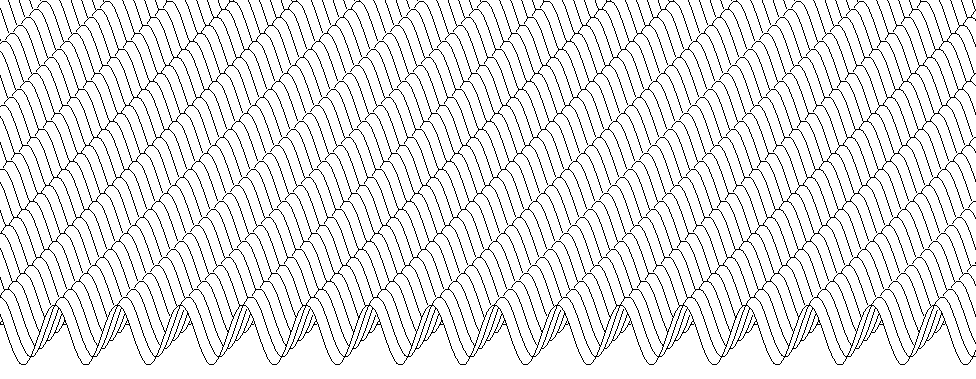And here’s another example with $z = sin(xy)$: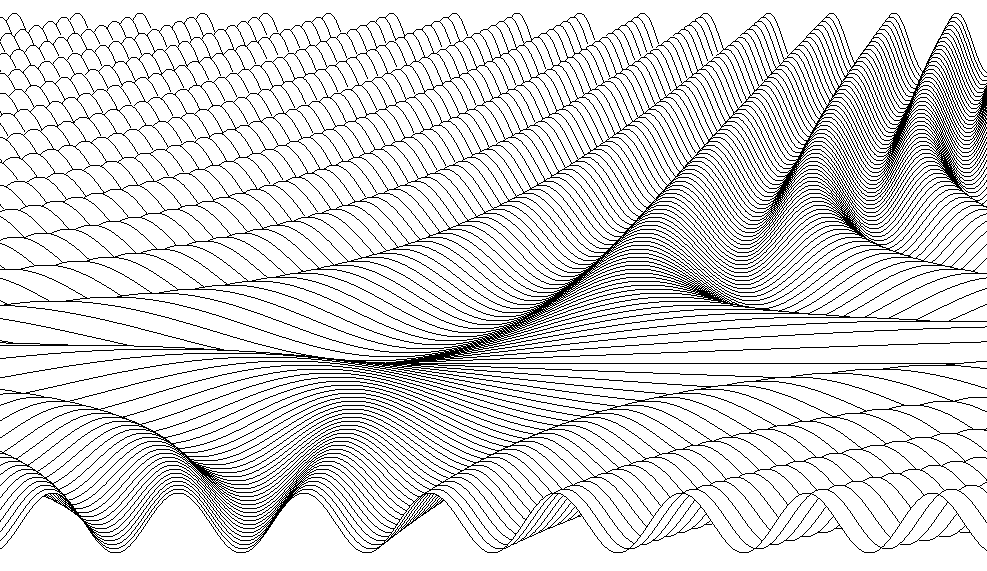We can also add some other eye-candy, like limiting the (X,Y) domain to a circle. Here $sin(x^2 + y^2)$ and $(x-y)e^{-(x^2 + y^2)}$: